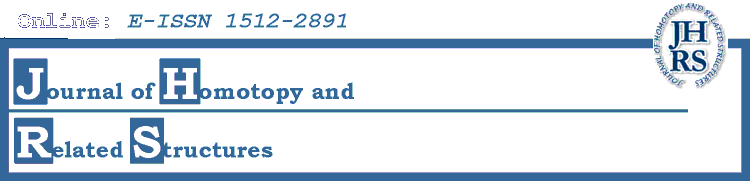# Volume 3 (2008), No. 1

1. A partial $A_\infty$-structure on the cohomology of $C_n\times C_m$

2. Cohomology of Categorical Self-Distributivity

3. Characteristic classes of $\ai$-algebras

4. Formal Homotopy Quantum Field Theories, I: Formal Maps and Crossed $\mathcal{C}$-algebras.

5. Generalized André-Quillen Cohomology

6. The inner automorphism 3-group of a strict 2-group

7. Infinity-Inner-Products on A-Infinity-Algebras

8. Cubical cospans and higher cobordisms (Cospans in Algebraic Topology, III)

9. Simplicial resolutions and Ganea fibrations

10. Exact sequences of fibrations of crossed complexes, homotopy classification of maps, and nonabelian extensions ofgroups

11. Homotopy Inner Products for Cyclic Operads

12. The functors Wbar and Diag o Nerve are simplicially homotopy equivalent

13. A conjectured lower bound for the cohomological dimension of elliptic spaces

14. Higher Derived Brackets and Deformation Theory I